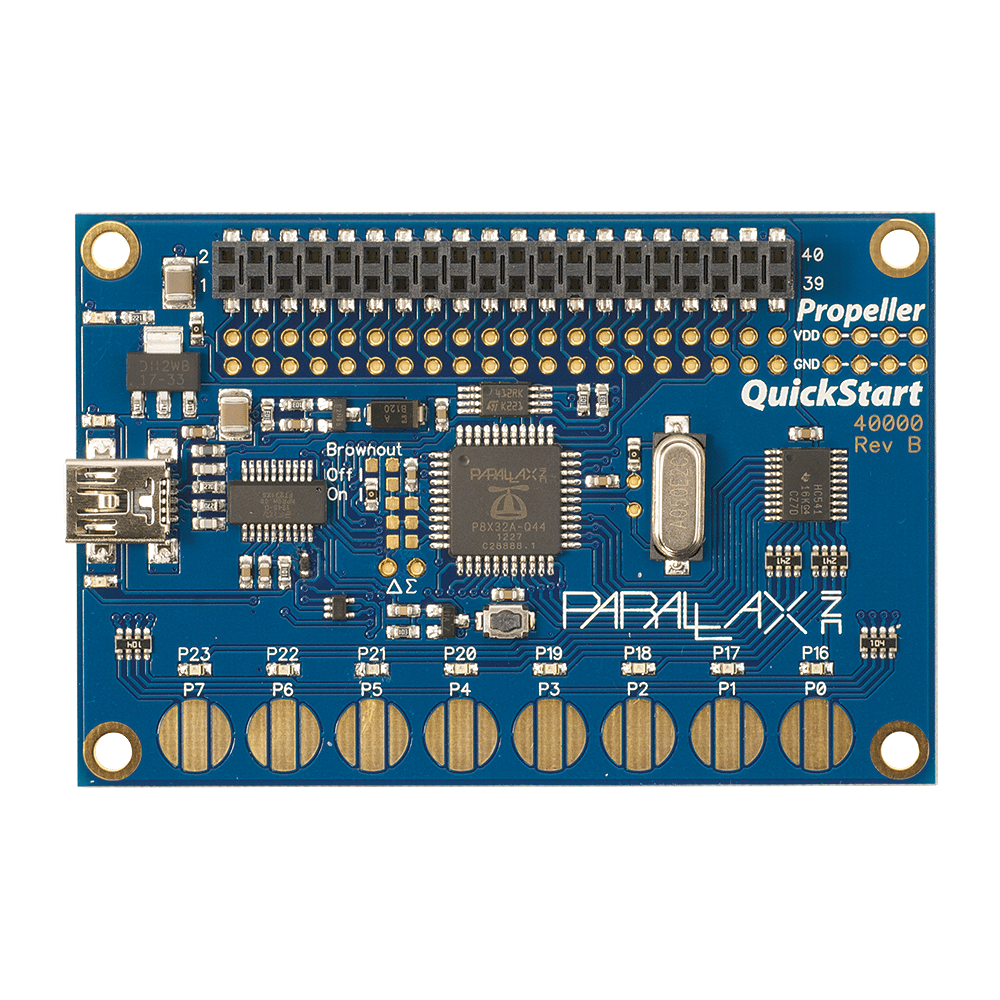PWM Measurement — Parallax Forums

# PWM Measurement

edited 2021-04-08 13:21

Hello my friends,

I have a problem with the PWM measurement. I cannot on my parallax card.

scenario:

We have 3 different PWM inputs and we want to measure them separately. How can I write this code? We need to see how many milliseconds are 1 and how many milliseconds are 0.• Here's a very simplistic approach:

```pub measure_cycle(pin, p_high, p_low) | mask, t0, t1, t2

dira[pin] := 0

if (ina[pin] == 0)
t0 := cnt
t1 := cnt
t2 := cnt
long[p_high] := (t1 - t0) / MS_001
long[p_low]  := (t2 - t1) / MS_001

else